Using Customer Lifetime Value in your business decision making is often important and crucial for success. Businesses that are customer-centric often spend thousands of dollars acquiring new customers, “on-boarding” new customers, and retaining those customers. If your business margins are thin, then it can often be months or quarters before you start to turn a profit on a particular customer. Additionally, some business models will segment the worth of their customers into categories that will often give different levels of service to the more “higher worth” customers. The metric most often used for that is called Customer Lifetime Value (CLV). CLV is simply a balance sheet look at the total cost spent versus the total revenue earned over a customer’s projected tenure or “life.”

In this blog, we will focus on how a business analyst can build a functional analytical dashboard for a fictional company that is seeing its revenue, margins, and a customer’s lifetime value decrease and what steps they can take to correct that.

We will cover 3 main areas of interest:

1. First, screenshots of SAS Visual Analytic reports, using Customer Lifetime Value and how you can replicate them.
2. Next, we will look at the modeling that we did in the report, with explanations on how we got used the results in subsequent modeling.
3. Lastly, we talk about one example of how we scored and deployed the model, and how you can do the same.

Throughout this blog, I will also highlight areas where SAS augments our software with artificial intelligence to improve your experience.

## 1. State of the company

First, we will look at the state of the company using the dashboard and take note of any problems.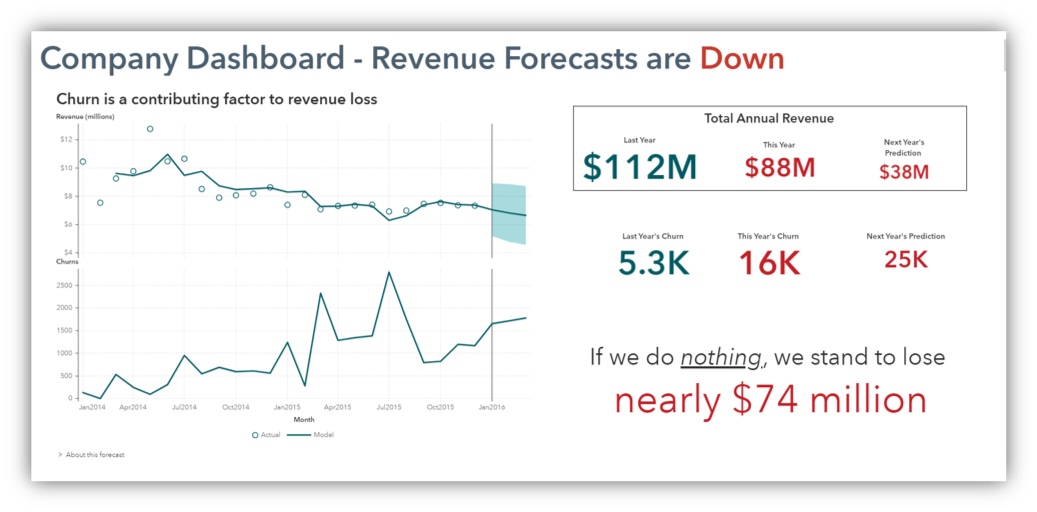Our dashboard shows the revenue of our company over the last two years as well as a forecast for the next 6 months. We see that revenue has been on the decline in recent years and churns have been erratically climbing higher.

Our total annual revenue was 112M last year with just over 5,000 customers churning.

So far this year, our revenue is tracking low and sits at only 88M, but the bad news is that we have already tripled last year's churn total.

If these trends continue, we stand to lose a third of our revenue!

## 2. The problems

Now, let’s investigate as to where the problems are and what can be done about them.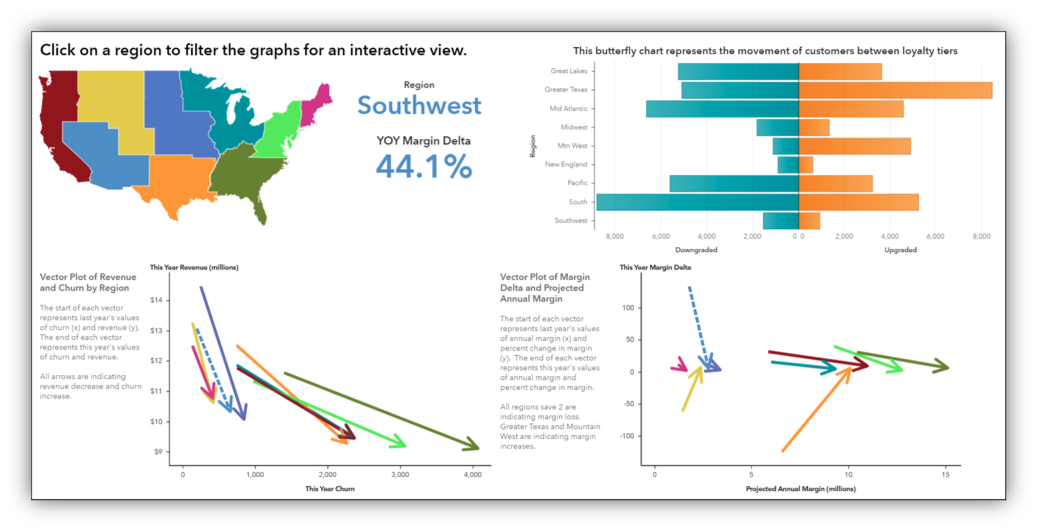If we look at our current metrics, we can see some interesting points worth investigating.

The butterfly chart on the right shows movement between customer loyalty tiers within each region of the country with the number of upgrades (on the right) and downgrades (on the left).

The vector plots show us information over multiple dimensions. These show us the difference between two values and the direction it is heading. For example, on the left, we see that Revenue is pointed downward while churns (x axis) are increasing.

The vector plot on the right shows us the change in margin from year to year as well as the customer lifetime value.

What’s interesting here is that there are two arrows that are pointing up, indicating a rise in customer lifetime value. Indeed, if we were to click on the map, we would see that these two regions are the same two that have a net increase in Loyalty Tier.

This leads me to believe that a customer’s tier is predictive of margin. Let’s investigate it further.

## 3. Automated Analysis

We will use the Automated Analysis feature within Visual Analytics to quickly give us the drivers of CLV.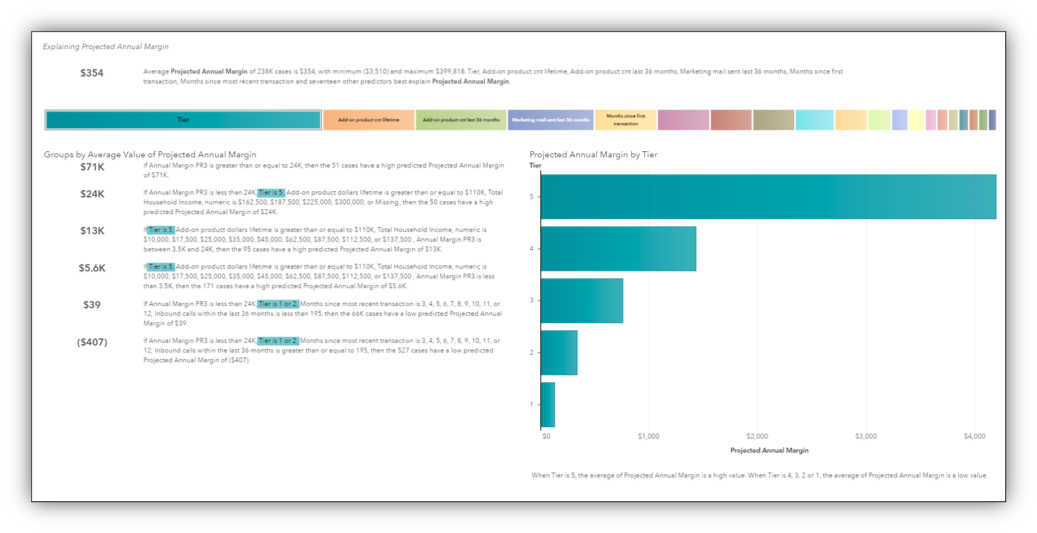This screenshot shows an analysis that SAS Visual Analytics(VA) performed for me automatically. I simply told VA which variable I was interested in analyzing and within a matter of seconds, it ran a series of decision trees to produce this summary. This is an example of how SAS is incorporating AI into our software to improve your experience.

Here we can see that loyalty tier is indeed the most important factor in determining projected annual margin (or CLV).

## 4. Influential driver

Once identified, the important driver will be explored across other dimensions to assess how influential this driver might be.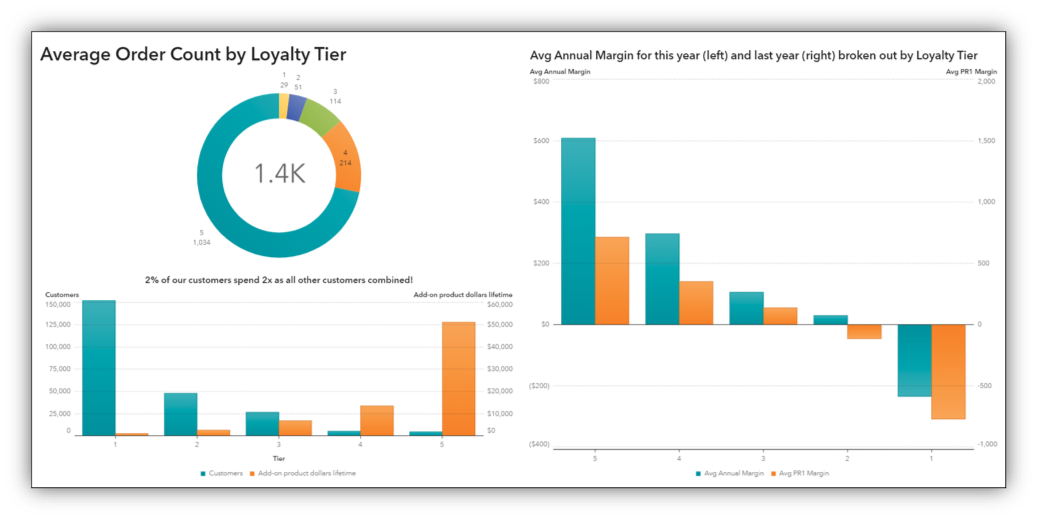A cursory exploration of Loyalty Tier indicates that yes, loyalty tier, particularly Tier 5, has a major influence on revenue, order count, repeat orders, and margin.

## 5. CLV comparison models

We will create two competing models for CLV and compare them.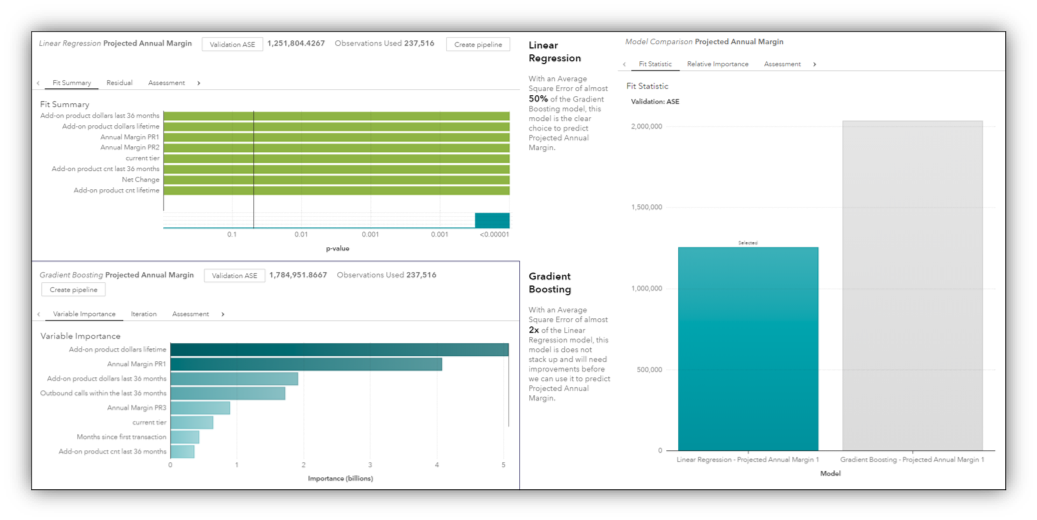Here on our modeling page are two models that I’ve created to predict CLV. The first one is a Linear Regression and the second is a Gradient Boosting model. I've used Model Comparison to tell me that the Linear Regression model delivers a more accurate prediction and so I use the output of that model as input into a recommendation engine.

## 6. Recommendation engine

Based on our model learnings and the output of the model, we are going to build a recommendation engine to help us with determine what to do with each customer.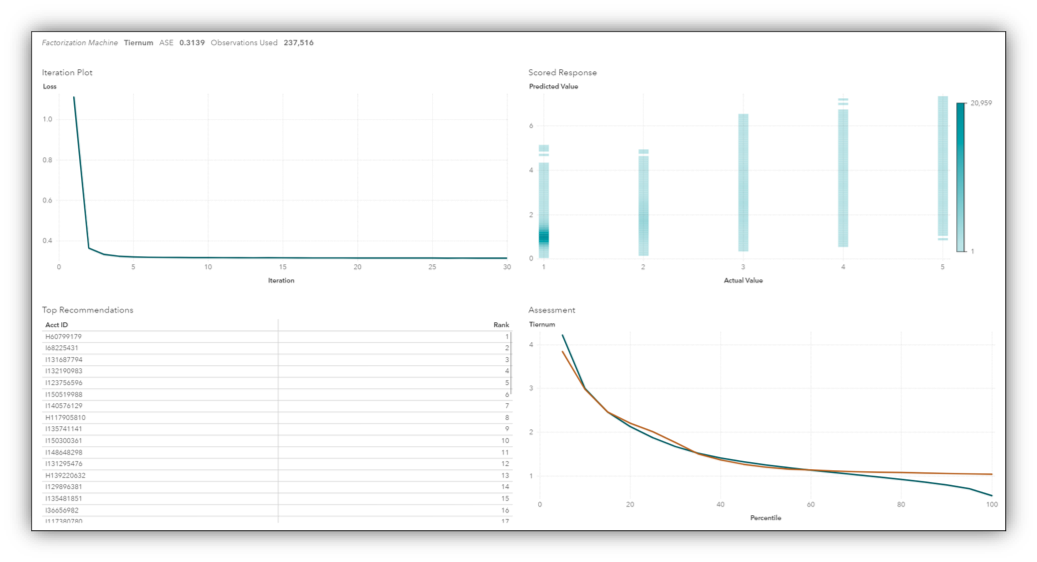Represented here, I built a recommendation engine model using the Factorization Machine algorithm.

Once we implement our model, customers are categorized more appropriately and we can see that it has had an impact on revenue and the number of accounts is back on the rise!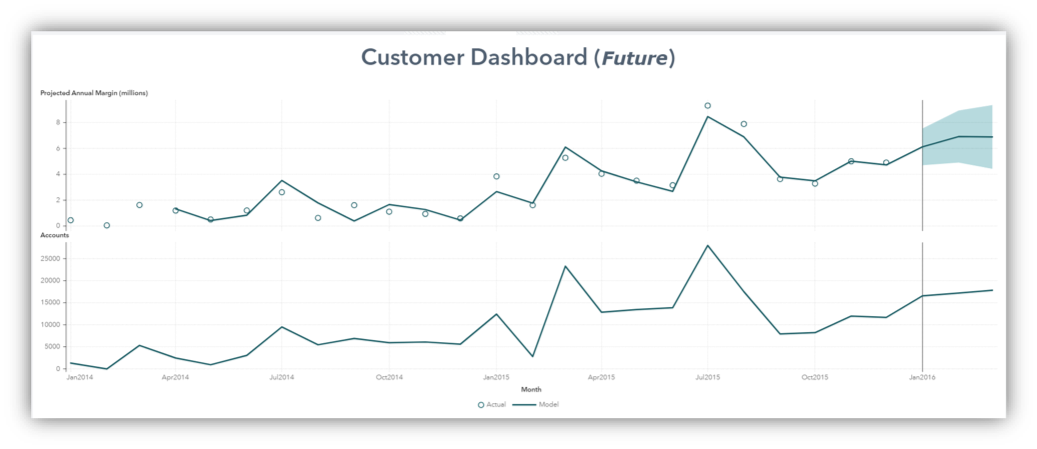## Conclusion

Even though Customer Lifetime Value has been around for years, it is still a valuable metric to utilize in modeling and recommendation engines as we have seen. We used it our automated analysis, discovered that it had an impact on revenue, we modeled future values of CLV and then incorporated those results into a recommendation engine that recommended new loyalty tiers for our customers. As a result, we saw positive changes in overall company revenue and churn.The title of this post borrows from Stanley Kubrick’s 1964 comedy “Dr. Strangelove or: How I Learned to Stop Worrying and Love the Bomb.” It stars the great Peter Sellers as the titular character as well as George C. Scott and Slim Pickens. The black and white film is strange and brilliant just like Kubrick was. Similarly, as I was experiencing the issue I outline below and was told of this solution, I thought two things. The first was “what a strange but brilliant solution” and the second one was “I’ll try anything as long as it works.”   Thus, a title was born. But enough about that. Why are we here?

### Problem

You want to add a couple of columns of information to your already large dataset, but each time you try to join the tables you run out of memory!  For example, you want to append latitude and longitude values from Table B to an existing list of customer phone numbers in Table A.

You’ve tried this and got nowhere fast:

```proc sort data = demo.tablea; by npa nxx; proc sort data = demo.tableb; by npa nxx; run;   data demo.aunionb; merge demo.tablea (in=a) demo.tableb (in=b); by npa nxx; if a; run;```

And then you tried this and also got nowhere (albeit a little slower):

```proc sql; create table demo.aunionb as select *, from demo.tablea a left join demo.tableb b on (a.npa = b.npa) and (a.nxx = b.nxx); quit;```

### Solution - Joining tables with PROC FORMAT

Use PROC FORMAT!

Here’s how:

First, take Table B and create character equivalents of the fields required in your join (assuming they aren’t characters already). In this example, NPA and NXX are the two fields that you are joining on. They will be your key once you concatenate them.  Next, create character equivalents of the fields that you want appended.

```data work.tableb (keep = npa_nxx--nxx_c); set demo.tableb;   npa_c = compress(put(npa, best10.)); nxx_c = compress(put(nxx, best10.));   npa_nxx = catx('_',npa_c, nxx_c);   lat_c = compress(put(latitude, best14.3)); long_c = compress(put(longitude, best14.3)); run;```

Next, make sure that you have only unique values of your key. Use PROC SORT with OPT=noduprecs turned on.

Now, create a table that will be used as the input into PROC FORMAT. In this example, you are creating a table that will contain the formats for the latitude column.

```proc sort data = work.tableb noduprecs; by npa_nxx;   data demo.tableb_lat_fmt(keep=fmtname type start label); retain fmtname 'lat_f' type 'C'; set work.tableb;   if npa_nxx = '._.' then start = 'Other '; else start = npa_nxx; label = lat_c; run; proc sort data = demo.tableb_fmt; by start; run;```

This step creates a table that includes the format name (lat_f), the format type (C), the key field (start) and its corresponding latitude value (label).  Sort this table by the ‘start’ column and then repeat this step for every column you wish to append, with each column getting its own unique format and table.

Now run PROC FORMAT using the CNTLIN option pointing to the tables that you just created in order to create your format.

```proc format cntlin=demo.tableb_lat_fmt; run; proc format cntlin=demo.tableb_long_fmt; run;```

Now all you have to do is run your data step to create the resultant dataset with the appended values.

```data demo.aunionb (drop = npa_nxx); set demo.tablea;   npa_nxx = catx('_',compress(put(npa,best10.)),compress(put(nxx, best10.)));   latitude = input(put(npa_nxx, \$lat_f.), BEST.); longitude = input(put(npa_nxx, \$long_f.), BEST.);   run;```

This step creates 3 columns: npa_nxx, latitude, and longitude. Npa_nxx is the key built from the NPA  and NXX values. Latitude and longitude are then populated with the formatted value of npa_nxx, which in this case is the character equivalent of the original latitude or longitude. It also formats the value back into a numeric field.

The result is a clever way to add columns to a dataset, much like a VLOOKUP function works in Microsoft Excel, without the hassle of running out of memory space.

### Notes:

1. The author realizes there are other, more boring ways of tackling this issue like indexing and using WHERE statements, but where’s the fun in that?
2. This solution may not be right for you. See your doctor if you experience any of the following symptoms:  memory loss, headache, frustration, Cartesian rage, cranial-keyboard embedment or memory loss.Recently, one of sons came to me and asked about something called “The Monty Hall Paradox.” They had discussed it in school and he was having a hard time understanding it (as you often do with paradoxes).

For those of you who may not be familiar with the Monty Hall Paradox, it is named for the host of a popular TV game show called “Let’s Make a Deal.” On the show, a contestant would be selected and shown a valuable prize.  Monty Hall would then explain that the prize is located just behind one of three doors and asked the contestant to pick a door.  Once a door was selected, Monty would then tease the contestant with cash to get him/her to either abandon the game or switch to another door.  Invariably, the contestant would stand firm and then Monty would proceed to show the contestant what was behind one of the other doors.  Of course, it wouldn’t be any fun if the prize was behind the revealed door, so after showing the contestant an empty door Monty would then ply them with even more cash, in the hopes that they would abandon the game or switch to the remaining door.

Almost without fail, the contestant would stand firm in their belief that their chosen door was the winner and would not switch to the other door.

When left with two doors, most people assume that they've got a 50/50 chance at winning. However, the truth is that the contestant will double his/her chance of winning by switching to the other door.

After explaining this to my son, it occurred to me that this would be an excellent exercise for coding in Python and in SAS to see how the two languages compared. Like many of you reading this blog, I’ve been programming in SAS for years so the struggle for me was coding this in Python.

I kept it simple. I generated my data randomly and then applied simple logic to each row and compared the results.  The only difference between the two is in how the languages approach it.  Once we look at the two approaches then we can look at the answer.

### First, let's look at SAS:

```data choices (drop=max); do i = 1 to 10000; u=ranuni(1); u2=ranuni(2); max=3; prize = ceil(max*u); choice = ceil(max*u2); output; end; run;```

I started by generating two random numbers for each row in my data. The first random number will be used to randomize the prize door and the second will be used to randomize the choice that the contestant makes. The result is a dataset with 10,000 rows each with columns ‘prize’ and ‘choice’ to represent the doors.  They will be random integers between 1 and 3.  Our next task will be to determine which door will be revealed and determine a winner.

If our prize and choice are two different doors, then we must reveal the third door. If the prize and choice are the same, then we must choose a door to reveal. (Note: I realize that my logic in the reveal portion is somewhat flawed, but given that I am using an IF…ELSE IF and the fact that the choices are random and there isn’t any risk of introducing bias, this way of coding it was much simpler.)

```data results; set choices; by i;   if prize in (1,2) and choice in (1,2) then reveal=3; else if prize in (1,3) and choice in (1,3) then reveal=2; else if prize in (2,3) and choice in (2,3) then reveal=1;```

Once we reveal a door, we must now give the contestant the option to switch. Switch means they always switch, neverswitch means they never switch.

```if reveal in (1,3) and choice in (1,3) then do; switch = 2; neverswitch = choice; end; else if reveal in (2,3) and choice in (2,3) then do; switch = 1; neverswitch = choice; end; else if reveal in (1,2) and choice in (1,2) then do; switch = 3; neverswitch = choice; end;```

Now we create a column for the winner.  1=win 0=loss.

``` switchwin = (switch=prize); neverswitchwin = (neverswitch=prize); run;```

Next, let’s start accumulating our results across all of our observations.  We’ll take a running tally of how many times a contestant who switches win as well as for the contestant who never switches.

```data cumstats; set results; format cumswitch cumnever comma8.; format pctswitch pctnever percent8.2; retain cumswitch cumnever; if _N_ = 1 then do; cumswitch = 0; cumnever = 0; end; else do; cumswitch = cumswitch+switchwin; cumnever = cumnever+neverswitchwin; end;   pctswitch = cumswitch/i; pctnever = cumnever/i; run;   proc means data=results n mean std; var switchwin neverswitchwin; run; legend1 frame ; symbol1 interpol=splines; pattern1 value=ms; axis1 style=1 width=1 minor=none ; axis2 style=1 width=1 major=none minor=none ; axis3 style=1 width=1 minor=none ; title; title1 " Cumulative chances of winning on Let's Make a Deal ";   proc gplot data=work.cumstats; plot pctnever * i / areas=1 frame vaxis=axis1 haxis=axis2 lvref=1 cvref=black vzero legend=legend1; plot2 pctswitch * i = 2 / areas=1 vaxis=axis3 vzero overlay legend=legend1 ; run; quit; ```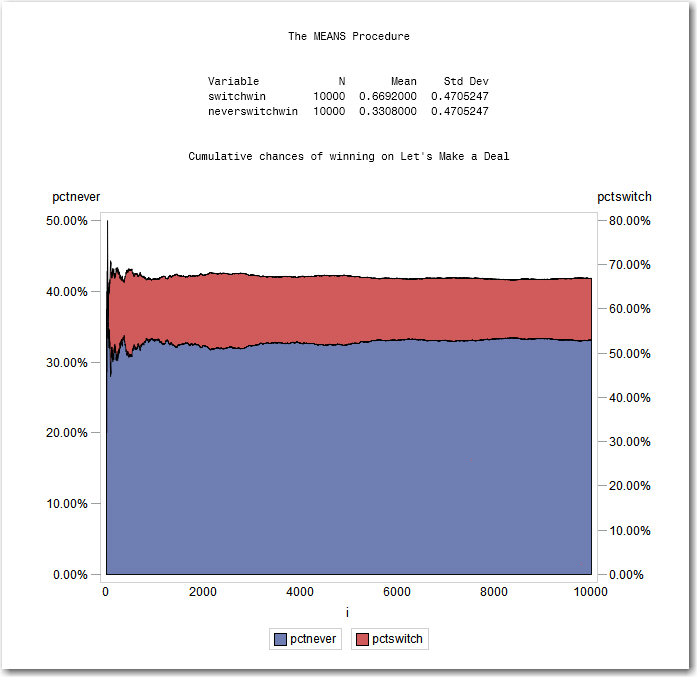The output of PROC MEANS shows that people who always switch (switchwin) have a win percentage of nearly 67%, while the people who never switch (neverswitchwin) have a win percentage of only 33%. The Area Plot proves the point graphically showing that the win percentage of switchers to be well above the non-switchers.

Now let’s take a look at how I approached the problem in Python (keeping in mind that this language is new to me).

### Now, let’s look at Python:

Copied from Jupyter Notebook

```import random import pandas as pd import numpy as np import matplotlib.pyplot as plt from itertools import accumulate %matplotlib inline```

First let's create a blank dataframe with 10,000 rows and 10 columns, then fill in the blanks with zeros.

```rawdata = {'index': range(10000)} df = pd.DataFrame(rawdata,columns=['index','prize','choice','reveal','switch','neverswitch','switchwin','neverswitchwin','cumswitch','cumnvrswt']) df = df.fillna(0)```

Now let's populate our columns. The prize column represents the door that contains the new car! The choice column represents the door that the contestant chose. We will populate them both with a random number between 1 and 3.

```prize=[] choice=[] for row in df['index']: prize.append(random.randint(1,3)) choice.append(random.randint(1,3)) df['prize']=prize df['choice']=choice```

Now that Monty Hall has given the contestant their choice of door, he reveals the blank door that they did not choose.

```reveal=[] for i in range(len(df)): if (df['prize'][i] in (1,2) and df['choice'][i] in (1,2)): reveal.append(3) elif (df['prize'][i] in (1,3) and df['choice'][i] in (1,3)): reveal.append(2) elif (df['prize'][i] in (2,3) and df['choice'][i] in (2,3)): reveal.append(1) df['reveal']= reveal```

Here's the rub. The contestant has chosen a door, Monty has revealed a blank door, and now he's given the contestant the option to switch to the other door. Most of the time the contestant will not switch even though they should. To prove this, we create a column called 'switch' that reflects a contestant that ALWAYS switches their choice. And, a column called 'neverswitch' that represents the opposite.

```switch=[] neverswitch=[] for i in range(len(df)): if (df['reveal'][i] in (1,3) and df['choice'][i] in (1,3)): switch.append(2) elif (df['reveal'][i] in (1,2) and df['choice'][i] in (1,2)): switch.append(3) elif (df['reveal'][i] in (2,3) and df['choice'][i] in (2,3)): switch.append(1) neverswitch = choice df['switch']=switch df['neverswitch']=neverswitch```

Now let's create a flag for when the Always Switch contestant wins and a flag for when the Never Switch contestant wins.

```switchwin=[] neverswitchwin=[] for i in range(len(df)): if (df['switch'][i]==df['prize'][i]): switchwin.append(1) else: switchwin.append(0) if (df['neverswitch'][i]==df['prize'][i]): neverswitchwin.append(1) else: neverswitchwin.append(0) df['switchwin']=switchwin df['neverswitchwin']=neverswitchwin```

Now we accumulate the total number of wins for each contestant.

```cumswitch=[] cumnvrswt=[] df['cumswitch']=list(accumulate(df['switchwin'])) df['cumnvrswt']=list(accumulate(df['neverswitchwin']))```

…and divide by the number of observations for a win percentage.

```pctswitch=[] pctnever=[] for i in range(len(df)): pctswitch=df['cumswitch']/(df['index']+1) pctnever=df['cumnvrswt']/(df['index']+1) df['pctswitch']=pctswitch df['pctnever']=pctnever```

Now we are ready to plot the results. Green represents the win percentage of Always Switch, blue represents the win percentage of Never Switch.

```x=df['index'] y=df['pctswitch'] y2=df['pctnever'] fig, ax = plt.subplots(1, 1, figsize=(12, 9)) ax.plot(x,y,lw=3, label='Always', color='green') ax.plot(x,y2,lw=3, label='Never',color='blue',alpha=0.5) ax.fill_between(x,y2,y, facecolor='green',alpha=0.6) ax.fill_between(x,0,y2, facecolor='blue',alpha=0.5) ax.set_xlabel("Iterations",size=14) ax.set_ylabel("Win Pct",size=14) ax.legend(loc='best') plt.title("Cumulative chances of winning on Let's Make a Deal", size=16) plt.grid(True)```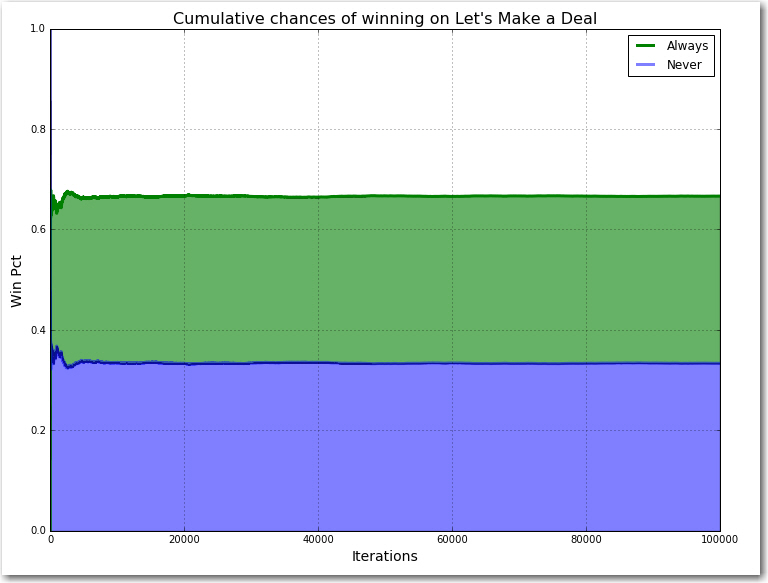### Why does it work?

Most people think that because there are two doors left (the door you chose and the door Monty didn’t show you) that there is a fifty-fifty chance that you’ve got the prize.  But we just proved that it’s not, so “what gives”?

Remember that the door you chose at first has a 1/3 chance of winning.  That means that the other two doors combined have a 2/3 chance in winning.  Even though Monty showed us what’s behind one of those two doors, the two of them together still have a 2/3 chance of winning.  Since you know one of them is empty, that means the door you didn’t pick MUST have a 2/3 chance of winning.  You should switch.  The green line in the Python graph (or the red line in the SAS graph) shows that after having run 10,000 contestants through the game the people that always switched won 67% of the time while the people that never switched only won 33% of the time.

### My comparisons and thoughts between SAS and Python.

In terms of number of lines of code required, SAS wins hands down.  I only needed 57 lines of code to get the result in SAS, compared to 74 lines in Python. I realize that experience has a lot to do with it, but I think there is an inherent verbosity to the Python code that is not necessarily there in SAS.

In terms of ease of use, I’m going to give the edge to Python.  I really liked how easy it was to generate a random number between two values.  In SAS, you have to actually perform arithmetic functions to do it, whereas in Python it’s a built-in function. It was exactly the same for accumulating totals of numbers. It was exactly the same for accumulating totals of numbers.  In Python, it was the accumulate function. In SAS, it was a do loop that summed each of the previous values.

In terms of iterative ability and working “free style,” I give the edge to SAS.  With Python, it is easy to iterate, but I felt myself having to start all over again having to pre-define columns, packages, etc., in order to complete my analysis.  With SAS, I could just code.  I didn’t have to start over because I created a new column.  I didn’t have to start over because I needed to figure out which package I needed, find it on Github, install it and then import it.

In terms of tabular output, SAS wins.  Easy to read, easy to generate.

In terms of graphical output, Python edges SAS out.  Both are verbose and tedious to get it to work. Python wins because the output is cleaner and there are way more options.

In terms of speed, SAS wins.  On my laptop, I could change the number of rows from 10,000 to 100,000 without noticing much of a difference in speed (0.25 – 0.5 seconds).  In Python, anything over 10,000 got slow.  10,000 rows was 6 seconds, 100,000 rows was 2 minutes 20 seconds.

Of course, this speed has a resource cost.  In those terms, Python wins.  My Anaconda installation is under 2GB of disk space, while my particular deployment of SAS requires 50GB of disk space.

Finally, in terms of mathematics, they tied.  They both produce the same answer as expected.  Of course, I used extremely common packages that are well used and tested.  Newer or more sophisticated packages are often tested against SAS as the standard for accuracy.

But in the end, comparing the two as languages is limited.  Python is much a more versatile object oriented language that has capabilities that SAS doesn’t have.  While SAS’ mature DATA step can do things to data that Python has difficulty with.   But most importantly, is the release of SAS Viya. Through Viya’s open APIs and micro-services, SAS is transforming itself into something more than just a coding language, it aims to be the analytical platform that all data scientists can use to get their work done.

tags: Python, SAS Programmers

The Monty Hall Paradox - SAS vs. Python was published on SAS Users.